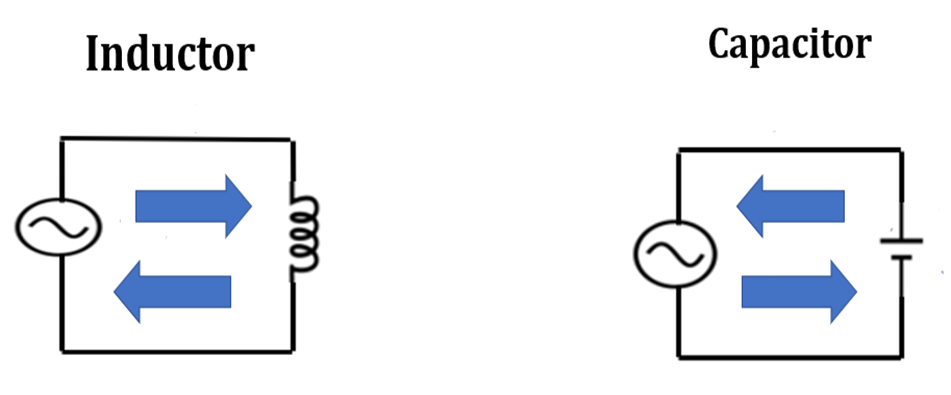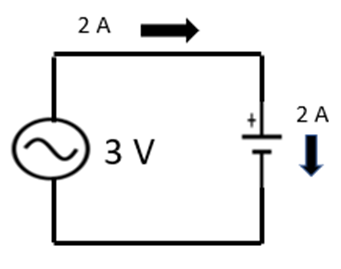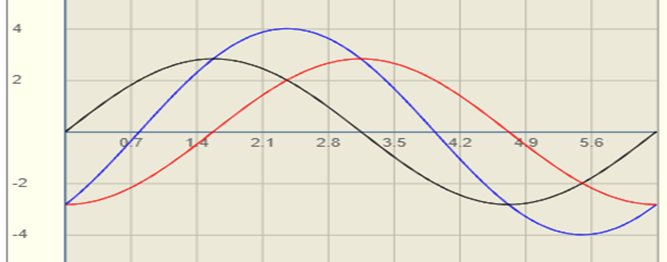top of page

# How Distribution Capacitor Banks Compensate for Inductive Loads

I’ve developed two separate explanations of this topic in an effort to reach the two audiences I’ve encountered over the years who seem to be interested in understanding it – Technical and Non-Technical people.

I start, below, with the simpler version, entitled “Plain Talk”, which is intended for people with no technical background in electricity. For those looking to dig a little deeper, a more in-depth explanation follows. Entitled “Working Knowledge”, it goes into some of the details much more deeply and is intended for people who DO have a technical background in electricity.

If you'd like to check them out, I’ve put videos for both on YouTube under the same “How Distribution Capacitor Banks Compensate for Inductive Loads” title.

### "Plain Talk" Version

To get started, we’ll look at three types of loads that are connected to electric distribution circuits to learn why Electric Utilities use capacitors. This explanation uses my “mathless” approach to the topic with simple diagrams to illustrate what’s happening.

Let’s start with the most common type of residential loads which we’ll refer to as “Active”. They’re also called Resistive or Real and there are many different examples of this type of load. In this simple circuit diagram, we have just one Source and one active load connected together.The Source delivers electrical energy to the circuit and the active load removes that electrical energy from the circuit and converts it to some other useful form of energy. In this case, we’ll say that the active element is a water heater converting electrical energy to heat to produce hot water.

I’ve placed a Blue Arrow in the middle of our circuit to depict this continuous one-way flow of energy from the source to the load.

The next category of loads is called “Reactive” and there are only two types, shown here – Inductors and Capacitors.True to their name, reactive loads spend half of their time performing an “Action” - removing energy from their circuit and storing that energy in their fields (a magnetic field for the inductor and an electric field for the capacitor) – and the other half of their time performing their “Reaction” - returning that stored energy back to their circuits. Unlike active devices, these reactive devices only temporarily remove and store energy from their circuits and, then, return that energy back to those circuits so, at the end of this “cycle”, the net transfer of energy between the Source and the reactive load is zero because all the energy delivered to the circuit by the source is removed, stored and, then, simply returned back to the source by the reactive load.

Again, I’ve placed Blue Arrows in the middle of these circuits to depict this “exchange” but, please note, two important points about these arrows:

1. The arrows point in both directions in each circuit to account for the flow of energy during both of their “Action” and “Reaction” phases.

2. The upper (and lower) blue arrows in the two circuits point in opposite directions. This is done to show that, in real time (when they’re in the same circuit together), their actions are exactly opposite one another – so, for example, when the inductor is removing energy from its circuit, the capacitor is returning energy to its circuit and vice versa. We’ll soon see how this out of sync activity can be used to our advantage.

Our last category of Load is comprised of loads that are a combination of the first two categories - Active and Reactive. Motors, which are a combination of resistive and inductive loads, represent the largest group of this type of load. They are different from our first two categories simply because they are a combination of both of them – drawing both Active and Reactive power. We’ll use an elevator motor as our example because it uses reactive power to create the magnetic fields that allow it to spin and it uses active power to lift people from one floor to the next.Let’s look at some feeder diagrams to discuss the nuances of these different loads. To start, we’ll look at a simplified diagram (below) representing the distribution feeder serving our Active, water heater customer.In real-life, this feeder would be a few miles long and serve hundreds of customers but, for our purposes, here, we’ll just show our water heater customer’s pole and imagine that it’s near the end of the circuit. It draws only active power, so, that energy must be supplied by the source feeding its substation and has to flow the full length of the feeder, all the way to the customer’s load as we can see here.

An important point to be made here is that the current flowing through the feeder’s conductors generates heat in those conductors which is lost into the air.Perhaps surprisingly, these “losses” represent the largest losses on a Utility’s electric system. In the case of active loads, there’s nothing that can be done to minimize these losses other than properly sizing the conductors.

Now let’s look at our customer with the elevator motor load…To simplify our comparison with the water heater customer, we’ll say that the motor is drawing the same amount of real power as the water heater and, also, that same amount of reactive power too. Again, this combination of active and reactive current flows from the substation all the way down the feeder to the customer. Surprisingly, however, the losses from conductor heating in this circuit will be much more than twice as much as the losses in the water heater circuit.

Luckily, in this case, however, the losses caused by the reactive current flow can be “compensated”. Thinking back to our conversation about Inductors and Capacitors, we’ve actually already discussed the solution to these losses – we’ll just put a capacitor with the same reactive value as the motor’s reactive draw right on the pole outside this customer’s facility.

In the diagram below, that’s exactly what we’ve done – we’ve added a capacitor on the customer’s pole that “Compensates” for the motor’s reactive draw.Because the capacitor and inductor are the same size, when the customer’s inductor is removing energy from the circuit to store in its field, the capacitor is returning that same amount of energy back to the circuit, and vise versa, so they simply “exchange” their stored energies, back and forth, and this leaves the source only having to feed just the active motor load. This means that the source is supplying the minimum amount of energy (the active amount) to the circuit and the losses are minimized to the same amount as in the water heater circuit.

Summary

While it may seem like a small point, at first, the result of adding the capacitors to compensate for inductive load is the elimination of the losses that the extra reactive current wastes in the conductors and is, perhaps surprisingly, a huge savings for the utility.

So, to summarize, conductor losses are a Utility’s greatest source of losses on their systems and, while the conductor losses created by active power can’t be compensated, using capacitors to compensate for reactive conductor losses represents a completely, cost effective method of improving their system’s efficiency.

### Introduction

Capacitors are used in Electric Utility T & D Systems to “compensate” for the extra current load of inductive devices such as motors and transformers. On distribution feeders, the effects of that current are two-fold - causing greater line losses and greater voltage drop - both of which decrease the system’s overall efficiency. Using properly placed and sized capacitors, these effects can be reduced and even eliminated. This article discusses some of the finer points of their application in circuits with resistive and inductive loads in parallel.

### Background

The source voltage in a 60 Hertz AC circuit changes magnitude constantly and reverses its polarity every half cycle. In our 60 Hertz systems, it takes 1/60 of a second (about 16.67 milliseconds) to complete one cycle of sine wave voltage as shown below…The peak values of the sine wave are 1.414 (√2) times its “nominal” value which, actually has three different, common names: 1. RMS - Root Mean Square, 2. DC Equivalent (DCE) and 3. Effective. It’s worth mentioning that the Y-axis will usually be marked with just one set of units, but those numbers can be applied to multiple units: for example, when voltage, current and watts are all displayed on one graph, one set of numbers will apply to all three quantities. As for the X-axis, in electrical graphs, it always represents a measurement of time but, quite often, it will be labeled in “degrees” to facilitate the math (here, it’s in radians).

## Performance of RLC Elements in AC Circuits

### ResistorIf we connect a 1 ½ ohm resistor to a 3-volt AC source, it will draw 2 amperes ….Note that this circuit obeys Ohm’s Law at all times so, since the voltage is constantly changing, the current also changes in direct proportion. The result is that the current and voltage are “in phase”, meaning that the zero points and peaks occur at the same time.

### InductorWhen we connect a 1 ½ ohm inductor to the same AC source, it also draws 2 amperes but, as we can see in our diagram below, this current “lags” the applied voltage by one quarter of a cycle or 90 …Unlike the resistor’s “in phase” current flow, however, this delayed response by the inductor’s current is due to the inductor alternately storing energy in and releasing energy from its magnetic field.

### CapacitorWhen we look at the current waveform, below, for this capacitor circuit, we can see that the current actually “leads” the voltage by one quarter of a cycle or 90…In much the same way as the inductor, the capacitor’s leading current response is a result of it alternately storing energy in and releasing energy from its electric field.

## Combining Elements Together

### Resistor and Inductor in Parallel

Now let’s look at a resistor and inductor in parallel. This will simulate a motor load on the feeder by causing the source current to lag the applied voltage, as we’ll soon see …Here are the waveforms: IR is in phase with the voltage, but IL lags the voltage by a quarter of a cycle or 90° as we’ve already seen …Now, let’s look at the SUM, below, of our two currents: IT = IR + IL. Surprisingly, their total, IT, is 2.8 A NOT their algebraic sum of 2 + 2 = 4 AND it’s not in phase with either of the two currents but, rather, halfway between the two at 45°…The important point to see here, is that the “total” current (2.8 A) is more than the “Real” current (2 A) and that means that there are unnecessary losses in the circuit conductors and a larger voltage drop which adversely affects the circuit’s ability to serve its customers.

Let’s look at a diagram of the distribution feeder to get a better feel for what this means in the real-life circuit …As you can see, the current of 2.8 A flows from the substation to the customer and the line losses and voltage drop are bigger than they need to be because of that.

Now, let’s add a capacitor in parallel with our RL combination to see how it “compensates” for the inductor’s effect on the circuit.

Here’s what our new circuit looks like …… and here are the currents in each of the three legs: IR, IL and IC. Remember that IR is “in phase” with the voltage and note that we picked IC to be the same 2 A as IL …Examining the IL and IC currents in this graph, we can see that they’re 180° out of phase with each other. This makes sense because IL lags the source voltage by 90° and IC leads the source voltage by 90°, so they’re 180° from each other. From the source’s perspective, that means that they cancel each other but what it really means to the circuit, is that, ignoring losses, these two devices feed each other’s current, power and energy needs. That leaves the source only having to supply the resistor with current, power and energy, so its output drops to an, in phase, current of 2 amps.

This is shown in the circuit diagram below with the two arrows depicting, separately, the flow between the source & resistor and the inductor & capacitor.Looking at the feeder diagram, below, we can now see how this affects the conductor losses and voltage drop. On the feeder conductors, the current flow is now the minimum of 2 A so, both the circuit losses and voltage drop are minimized. On the customer’s service drop, however, the same 2.8 A are still flowing due to the “exchange” of current between the customer’s inductive load and our pole-mounted capacitor which add with the resistive flow.In summary then, while the capacitor “compensates” for the customer’s Reactive, inductive “load”, the source now supplies only the circuit’s minimum current requirement - the resistor’s Real power and energy needs which makes the source voltage and current “in phase” and the power factor 1.0. This reduction in current also minimizes the circuit’s conductor losses and voltage drop which improves the feeder’s efficiency and voltage regulation.

The extra good news is that, while we’ve used a resistor and inductor in parallel for this example, the same process works for a resistor and inductor in series too. In either case, adding a parallel capacitor, whose value is equal to the inductive load, drops the source’s current output to just the, in phase, value needed for the resistive load.

## Too Little, Too Much and Just Right

Interestingly enough, “compensation” is an EXACT science, in the sense that,” too little” is, actually, the same as “too much”. Remember that the inductive and capacitive currents are 180° out of phase and, therefore, “cancel” each other. So, if, in our above example, the capacitor drew only 1.5 A, instead of 2 A, there would have been 0.5 A of inductive current that wouldn’t have been “canceled”. That would have meant that 0.5 A gets added to the resistive current causing more losses and voltage drop. But if, instead, there were 2.5 A of capacitive current then 2 A of that would’ve been “cancelled” by the inductive current and that extra 0.5 A of capacitive current would be added to the resistive current. That would result in the same current flow on the distribution feeder and the same losses and voltage drop caused by the excess inductive current.

So, in general, whether it’s inductive or capacitive, excess reactive current causes line losses and voltage drop that’s undesirable. In the real world, it’s usually the inductive load that we’re “chasing” in an attempt to “compensate” with capacitors and keep those undesirable effects to a minimum. Those efforts tend to keep the power factor somewhere between 90 and 100 percent.